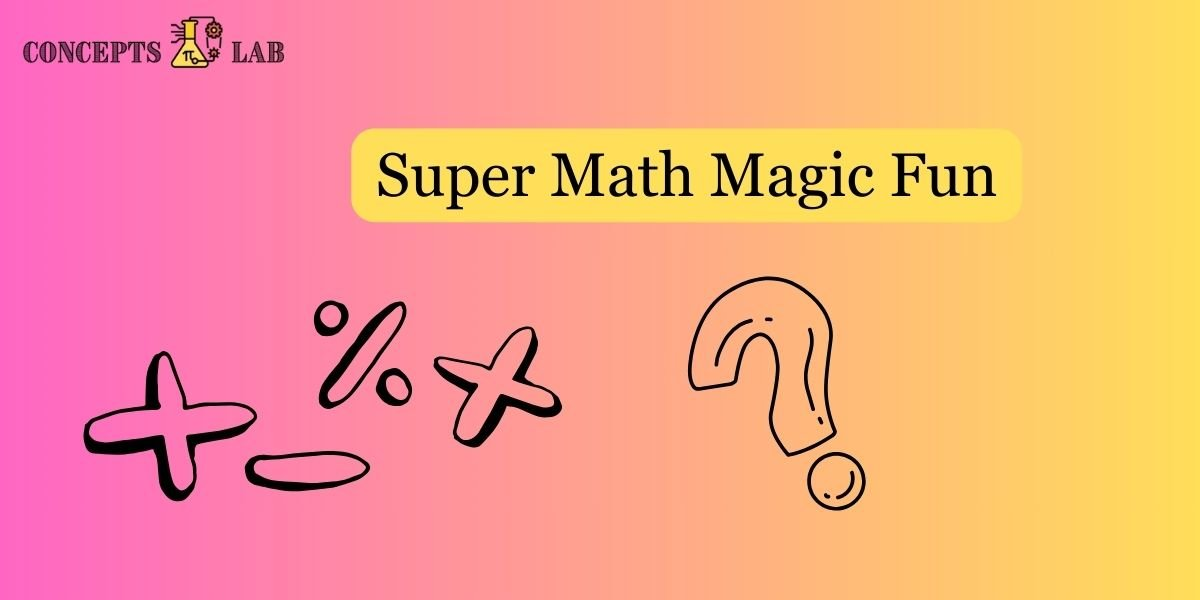Math magic involves using mathematical principles and tricks to create illusions, puzzles, and entertaining feats that seem almost like magic. Here are a few math magic tricks that you can try:

• Ask someone to choose any number. Multiply that number by 2, add 8, divide by 2, and subtract the original number. Regardless of the starting number, the result will always be 4.

### 2. Guess the Age:

• Ask someone to multiply their age by 2, add 8, divide by 2, and subtract their original age. The result is always 4.

### 3. Magical Multiplication:

• Ask someone to choose a number. Multiply it by 2, add 5, multiply by 3, subtract 7, and divide by 3. No matter what number they start with, the result is always 2.

### 4. Number Guessing:

• Have someone choose a number. Multiply it by 3, add 12, divide by 3, and subtract 4. The result will always be the number 4.

### 5. Magic Square:

• Create a 3×3 grid and ask someone to fill in any number. Then, complete the magic square by adding numbers in a way that each row, column, and diagonal adds up to the same number.

### 6. The 1089 Trick:

• Have someone choose a three-digit number with descending digits (e.g., 754). Reverse the digits (e.g., 457) and subtract the smaller number from the larger one. The result, when reversed and added to the original number, is always 1089.

### 7. Number Prediction:

• Write down a prediction number before the trick. Ask someone to choose a number, add 3, multiply by 2, subtract 4, divide by 2, and subtract the original number. Open your prediction, and it matches the result.

### 8. Calendar Magic:

• Ask someone to choose any date on the calendar. Multiply the day by 2, add 8, multiply by 5, add the month number, and subtract 4. You can reveal the day and month with this result.

Remember that these tricks rely on mathematical principles and clever arrangements, so the key is in understanding the math behind each trick. They can be a fun way to engage with math and surprise your friends with seemingly magical abilities.